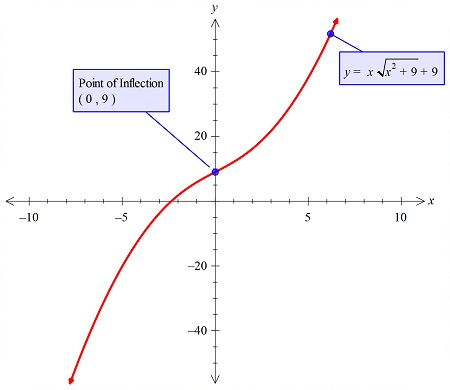# concave down in the intervals f(x) = xsqrt(x2+9)+9.

## Question:

Concave down in the intervals {eq}f(x) = x\sqrt{(x^2+9)}+9{/eq}.

## Graphs:

The point around which the curve is concave downward or concave upward is the inflection point. The curve that is concave upward is increasing and that is concave downward is decreasing.

## Answer and Explanation:

In the problem, we have to find the interval in which the function is concave down.

The fuction is:

{eq}f(x) = x\sqrt{(x^2+9)}+9 {/eq}

We will plot the graph of the function to find that, as the particular method is not metioned to use.

Thus the graph is:Thus the interval in which the graoh is concave down is:

{eq}(-\infty,0) {/eq}

#### Learn more about this topic:Graphs: Types, Examples & Functions

from High School Algebra II: Help and Review

Chapter 16 / Lesson 11
355K